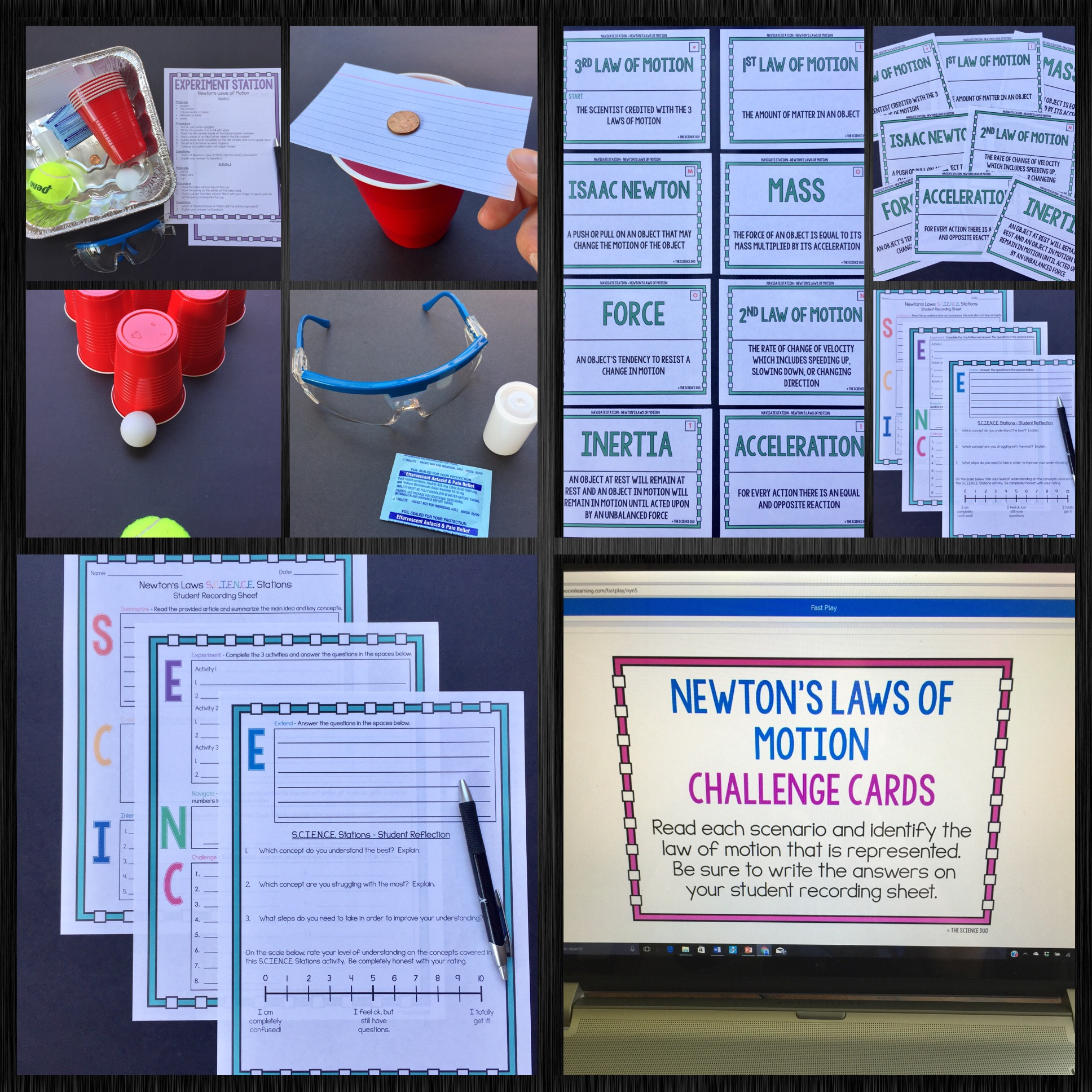### Force and Motion S.C.I.E.N.C.E. Station Lab Bundle

This Force and Motion S.C.I.E.N.C.E. Stations Bundle is designed to get your students engaged, collaborating, and moving in your daily lesson. This bundle includes 7 interactive and student-led Force and Motion S.C.I.E.N.C.E. Station labs. Each station provides a different method for reinforcing important science content. All stations correlate to a task that is represented by a letter in the word SCIENCE. These stations will have students Summarize, Create, Interpret, Experiment, Navigate, Challenge, and Extend.### Included in this Resource:

#### Check out the Details Below!

This Force and Motion Bundle includes the following science station labs:

•Average Speed S.C.I.E.N.C.E. Stations

•Motion Graphs S.C.I.E.N.C.E. Stations

•Net Force S.C.I.E.N.C.E. Stations

•Newton’s Laws S.C.I.E.N.C.E. Stations

•Speed, Velocity, and Acceleration S.C.I.E.N.C.E. Stations

•Work S.C.I.E.N.C.E. Stations

•Simple Machines S.C.I.E.N.C.E. Stations

Each S.C.I.E.N.C.E. Stations activity includes the following:
-Teacher Guide for implementing S.C.I.E.N.C.E. Stations (PRINT and READ FIRST)
-S.C.I.E.N.C.E. Station Signage
-General Directions for students
-Teacher Setup for specific stations
-7 station activities
-Student recording sheet and answer key
-Color and B&W options for all printable materials

Descriptions of the 7 S.C.I.E.N.C.E. Stations included are as follows:

Summarize – Students will read an article relating to a specific science concept. After reading the article, students will write a brief summary of the main ideas and key concepts contained in the article.

Create – Students will create a “graffiti wall” that will include words, phrases, pictures, diagrams, and/or icons to represent a specific science concept. Each student or group of students will add to the existing creation at this station.

Interpret – Students will interpret graphs, data tables, charts, diagrams, and/or pictures in order to answer a series of questions.

Experiment – Students will conduct a quick experiment or hands-on activity in order to demonstrate a specific science concept.

Navigate – Students will navigate through a card activity in order to decipher a code of symbols, numbers, and/or letters.

Challenge – Students will complete a challenge of digital BOOM Cards™ using computers, laptops, tablets, phones, or any electronic device with a modern web browser.

Extend – Students will extend their knowledge of a specific science concept. Students will answer questions to provoke deeper thought of the real world applications of science.

S.C.I.E.N.C.E. Stations Suggestions for Use
•    Use at the end of a unit of study to gauge student understanding
•    Use as an assessment activity
•    Use in tutorials or student pullouts for struggling learners
•    Use as a review or reteach activity
•    Use to increase overall student engagement